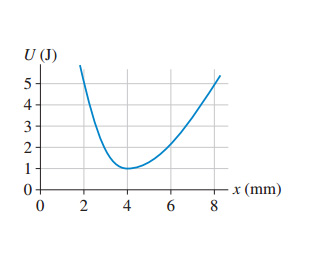# Problem: (a) What is the maximum speed of a 2.0 g particle that oscillates between x =2.0 mm and x =8.0 mm in ?(b) Estimate the force on the particle at x = 6.0 mm

###### FREE Expert Solution

In this problem, we are given a graph of potential energy against the position.

Force and potential energy are related by:

$\overline{){\mathbf{F}}{\mathbf{=}}{\mathbf{-}}\frac{\mathbf{∆}\mathbf{U}}{\mathbf{∆}\mathbf{x}}}$

(a)

From the graph, the particle has two turning points at 2mm and 8mm.

98% (185 ratings)###### Problem Details

(a) What is the maximum speed of a 2.0 g particle that oscillates between x =2.0 mm and x =8.0 mm in?

(b) Estimate the force on the particle at x = 6.0 mm

Frequently Asked Questions

What scientific concept do you need to know in order to solve this problem?

Our tutors have indicated that to solve this problem you will need to apply the Force & Potential Energy concept. You can view video lessons to learn Force & Potential Energy. Or if you need more Force & Potential Energy practice, you can also practice Force & Potential Energy practice problems.

What professor is this problem relevant for?

Based on our data, we think this problem is relevant for Professor Tolich's class at UW-SEATTLE.